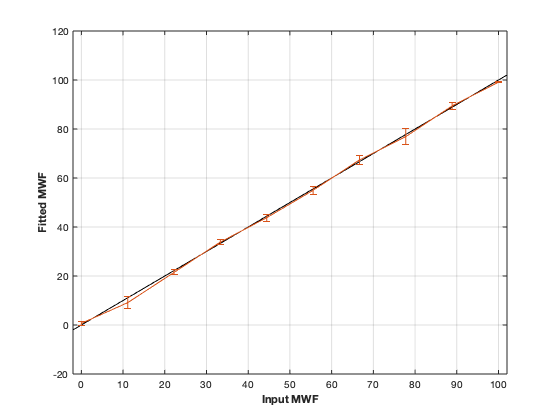# mwf : Myelin Water Fraction from Multi-Exponential T2w data¶## Contents

```% This m-file has been automatically generated using qMRgenBatch(mwf)
% Command Line Interface (CLI) is well-suited for automatization
% purposes and Octave.
%
% Please execute this m-file section by section to get familiar with batch
% processing for mwf on CLI.
%
%
% Written by: Agah Karakuzu, 2017
% =========================================================================
```

## I- DESCRIPTION

```qMRinfo('mwf'); % Describe the model
```
```  mwf :  Myelin Water Fraction from Multi-Exponential T2w data

Assumptions:

Inputs:
MET2data    Multi-Exponential T2 data

Outputs:
MWF       Myelin Wanter Fraction
T2MW      Spin relaxation time for Myelin Water (MW) [ms]
T2IEW     Spin relaxation time for Intra/Extracellular Water (IEW) [ms]

Options:
Cutoff          Cutoff time [ms]
Sigma           Noise standard deviation. Currently not corrected for rician bias
Relaxation Type
'T2'       For a SE sequence
'T2*'      For a GRE sequence

Protocol:
MET2data   [TE1 TE2 ...] % list of echo times [ms]

Example of command line usage:
Model = mwf;  % Create class from model
Model.Prot.MET2data.Mat=[10:10:320];
data = struct;  % Create data structure
FitResults = FitData(data,Model); %fit data
FitResultsSave_mat(FitResults);

For more examples: a href="matlab: qMRusage(mwf);"qMRusage(mwf)/a

Author: Ian Gagnon, 2017

References:
Please cite the following if you use this module:
MacKay, A., Whittall, K., Adler, J., Li, D., Paty, D., Graeb, D.,
1994. In vivo visualization of myelin water in brain by magnetic
resonance. Magn. Reson. Med. 31, 673?677.
In addition to citing the package:
Cabana J-F, Gu Y, Boudreau M, Levesque IR, Atchia Y, Sled JG,
Narayanan S, Arnold DL, Pike GB, Cohen-Adad J, Duval T, Vuong M-T and
Stikov N. (2016), Quantitative magnetization transfer imaging made
easy with qMTLab: Software for data simulation, analysis, and
visualization. Concepts Magn. Reson.. doi: 10.1002/cmr.a.21357

Reference page in Doc Center
doc mwf

```

## a- create object

```Model = mwf;
```

## b- modify options

```         |- This section will pop-up the options GUI. Close window to continue.
|- Octave is not GUI compatible. Modify Model.options directly.```
```Model = Custom_OptionsGUI(Model); % You need to close GUI to move on.
```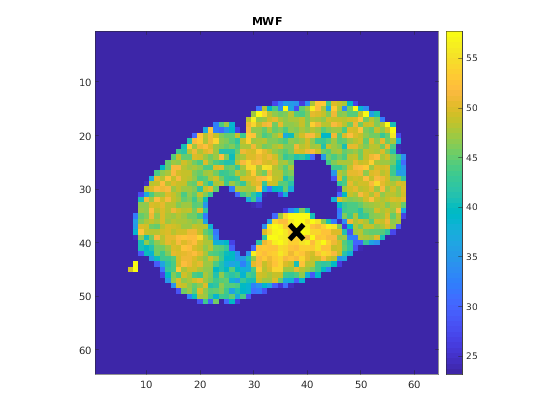## III- FIT EXPERIMENTAL DATASET

```         |- mwf object needs 2 data input(s) to be assigned:
|-   MET2data
```data = struct();

% MET2data.mat contains [64  64   1  32] data.
% Mask.mat contains [64  64] data.
data.MET2data= double(MET2data);
```

## b- fit dataset

`           |- This section will fit data.`
```FitResults = FitData(data,Model,0);
```
```Starting to fit data.
```

## c- show fitting results

```         |- Output map will be displayed.
|- If available, a graph will be displayed to show fitting in a voxel.
|- To make documentation generation and our CI tests faster for this model,
we used a subportion of the data (40X40X40) in our testing environment.
|- Therefore, this example will use FitResults that comes with OSF data for display purposes.
|- Users will get the whole dataset (384X336X224) and the script that uses it for demo
via qMRgenBatch(qsm_sb) command.```
```FitResults_old = load('FitResults/FitResults.mat');
qMRshowOutput(FitResults_old,data,Model);
```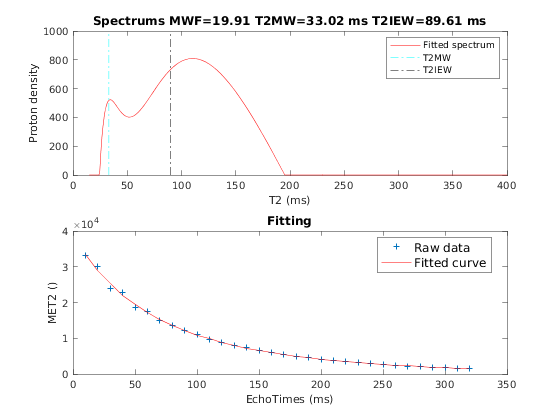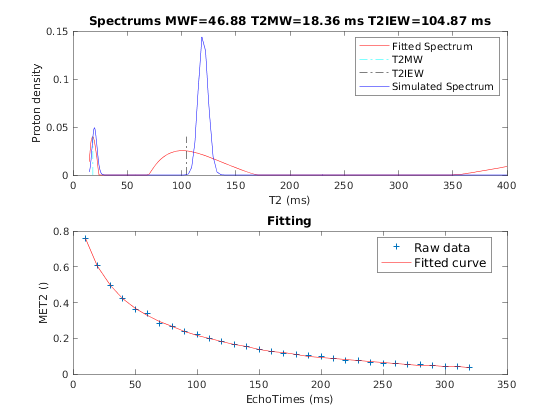## d- Save results

```         |-  qMR maps are saved in NIFTI and in a structure FitResults.mat
that can be loaded in qMRLab graphical user interface
|-  Model object stores all the options and protocol.
It can be easily shared with collaborators to fit their
own data or can be used for simulation.```
```FitResultsSave_nii(FitResults);
Model.saveObj('mwf_Demo.qmrlab.mat');
```
```Warning: Directory already exists.
```

## V- SIMULATIONS

`   |- This section can be executed to run simulations for mwf.`

## a- Single Voxel Curve

```         |- Simulates Single Voxel curves:
(1) use equation to generate synthetic MRI data
(3) fit and plot curve```
```      x = struct;
x.MWF = 50.0001;
x.T2MW = 20.0001;
x.T2IEW = 120;
% Set simulation options
Opt.SNR = 200;
Opt.T2Spectrumvariance_Myelin = 5;
Opt.T2Spectrumvariance_IEIntraExtracellularWater = 20;
% run simulation
figure('Name','Single Voxel Curve Simulation');
FitResult = Model.Sim_Single_Voxel_Curve(x,Opt);
```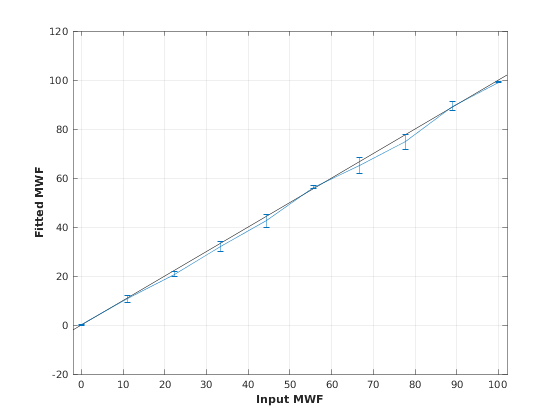## b- Sensitivity Analysis

```         |-    Simulates sensitivity to fitted parameters:
(1) vary fitting parameters from lower (lb) to upper (ub) bound.
(2) run Sim_Single_Voxel_Curve Nofruns times
(3) Compute mean and std across runs```
```      %              MWF           T2MW          T2IEW
OptTable.st = [50            20            1.2e+02]; % nominal values
OptTable.fx = [0             1             1]; %vary MWF...
OptTable.lb = [0.0001        0.0001        40]; %...from 0.0001
OptTable.ub = [1e+02         40            2e+02]; %...to 100
% Set simulation options
Opt.SNR = 200;
Opt.T2Spectrumvariance_Myelin = 5;
Opt.T2Spectrumvariance_IEIntraExtracellularWater = 20;
Opt.Nofrun = 5;
% run simulation
SimResults = Model.Sim_Sensitivity_Analysis(OptTable,Opt);
figure('Name','Sensitivity Analysis');
SimVaryPlot(SimResults, 'MWF' ,'MWF' );
```